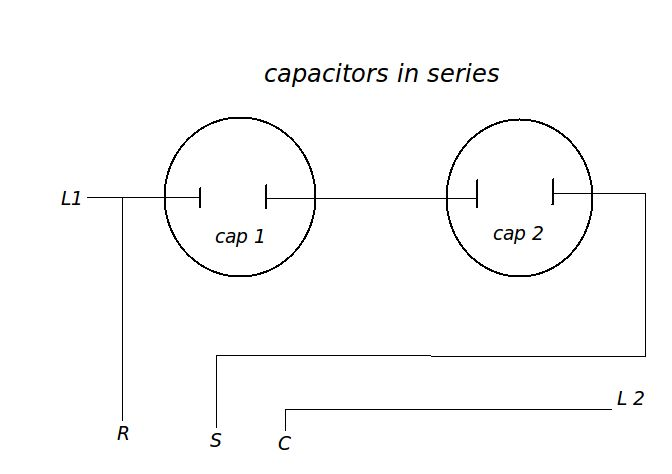# Motor and Capacitor Page

Testing a capacitor while running in the circuit
V= Voltage measured between run & start terminals
A = Amps measured at start wire going to compressor
Formula: uf = 2652 * Amps / Volts
Voltage
Amps
capacitors in series calculator

Capacitors in series can never be bigger than the smallest size in the series so a 5uf & 85uf will be smaller than 5uf.

Two of the same size capacitors in series equals 1/2 of the rating of one. So two 10uf caps = 5uf

 formula: uf= 1/ (1/cap1 + 1/cap2 ...) Cap 1: Cap 2: Cap 3:Enter any two numbers to get the third number
 Capacitor 1: Capacitor 2: Series uf=

Motor info: If you look at the diagram of a multi-speed motor, the speed taps actually all connect to the common motor winding.
For some good motor reading download Fasco's Fast Facts
 Amp rating : Ohms from Common to High : Common to Medium : Common to Low :This will find the amp ratings for lower speeds when only the high speed amp rating is given.
To find the lower amp ratings enter the motor amp rating in the first (amp rating) field.
Then enter the resistance for each corresponding speed.

 The formula: Multiply the max amp rating by the resitance from C to High. Take the result and divide it by the resistance measured from C to another speed. For instance you have a 3 speed motor with an amp rating of 2.9. You measure the resistance: C to High = 19 ohms    C to Med = 25 ohms     C to Low = 31 ohms. 19 x 2.9 = 55.1 now divide that by the different ohm readings you get from the different speeds. 55.1/25 = 2.20 amps med. 55.1/31 = 1.78 amps low